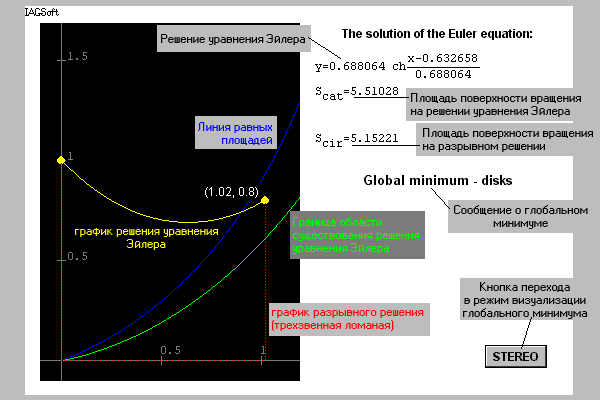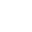Problem of the Minimum Rotation-Surface

It is necessary to find a generating line of the rotation-surface with minimum area. It is known that the line to be found is a catenary. Depending on the concrete boundary conditions, there can exist either two solutions of the Euler equation or alone or none. These solutions are catenaries. In traditional courses, one does not pay attention enough to the case of absence of the classic solution. In the numerical solution by the Euler method, the broken-wise solution is easily discovered. These are two disks for which the generating line consists of two vertical segments and a horizontal one lying on the axis of rotation. Moreover, the broken-wise solution can be a global minimum in the zone of existing the solution for the Euler equation (Ahiezer, 1955; Young, 1969; Cesari, 1983). If the left-end point is fixed at (0, 1), then one can construct a partition of the quadrant of feasible locations for the right-end point. If the right-end point is located in the left area (see Fig. 1), then the global minimum is a catenary. If this point is in the central area, then the global minimum is a broken-wise solution (disks) while the catenary gives only a local minimum. If the point is in the right area, then the only (global) minimum is the broken-wise solution, no solutions of the Euler equation exist. These facts can be found in (Anisimov, 1904; Ahiezer, 1955; Young, 1969; Cesari, 1983), however, they are not widely known.

 JAVA not supported!Fig. 1Press mouse button on black field

More accessible site

REFERENCES

Ahiezer, N.I. (1955). Lectures on the calculus of variations. TehGIZ, Moscow [in Russian].

Anisimov, V. (1904). Course on the calculus of variations. V. I, Warshaw [in Russian].

Cesari, L. (1983) Optimization Theory and Applications Problems with Ordinary Differential Equations. Springer-Verlag, N.Y., Heidelberg, Berlin.

Young, L.C. (1969). Lectures on the calculus of variations and optimal control theory. W.B. Saunders Co., Philadelphia, London, Toronto.iagsoft@imm.uran.ru